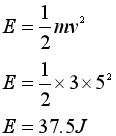# A 3kg book is tossed across the room at a speed of 5m/s. What is the Kinetic energy of the book? A.0.75 J B.3.75J C.15 J D.75J

Question
1 views
1. A 3kg book is tossed across the room at a speed of 5m/s. What is the Kinetic energy of the book?
 A. 0.75 J B. 3.75J C. 15 J D. 75J
check_circle

Step 1

Given

Mass m = 3 kg

Velocity v = 5 m/s

Step 2

Solution

Kinetic energy...

### Want to see the full answer?

See Solution

#### Want to see this answer and more?

Solutions are written by subject experts who are available 24/7. Questions are typically answered within 1 hour.*

See Solution
*Response times may vary by subject and question.
Tagged in